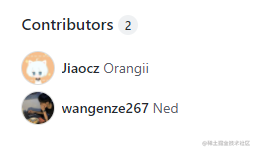我的第一次PR，一个炫酷的个人介绍页面个人简介页面模板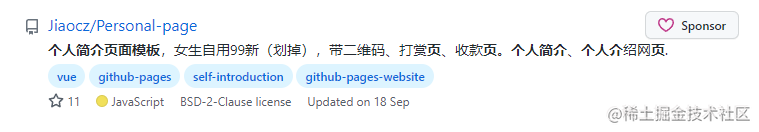女生自用99新？？？？引起了我极大的好奇心，连忙点进去。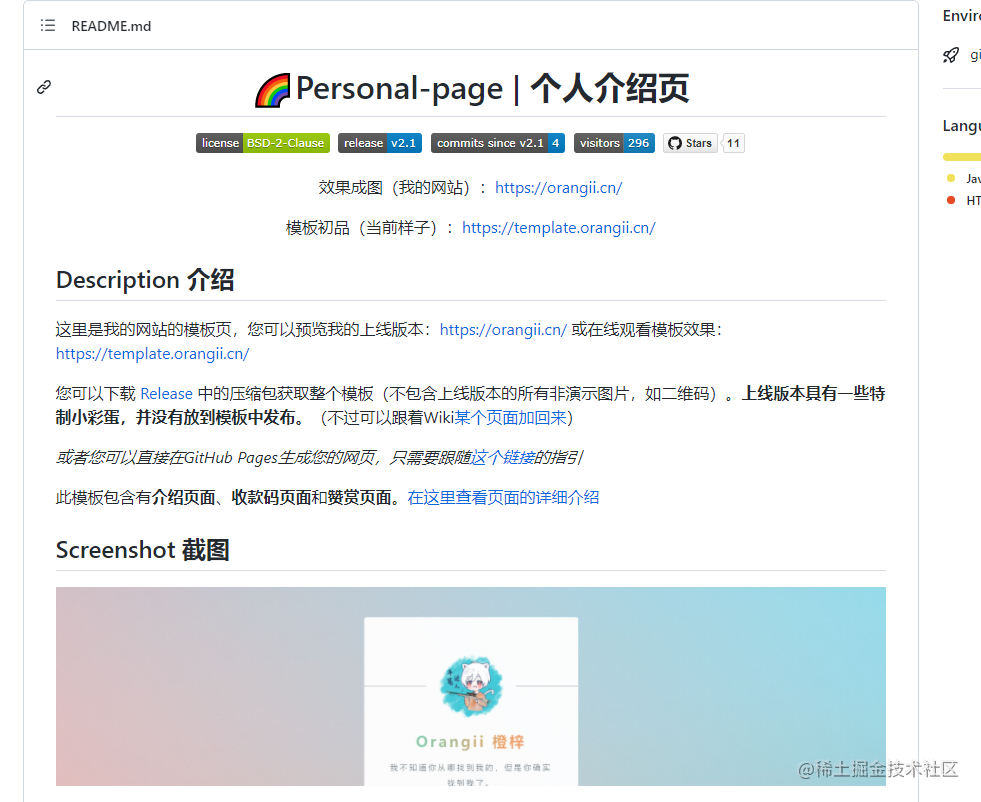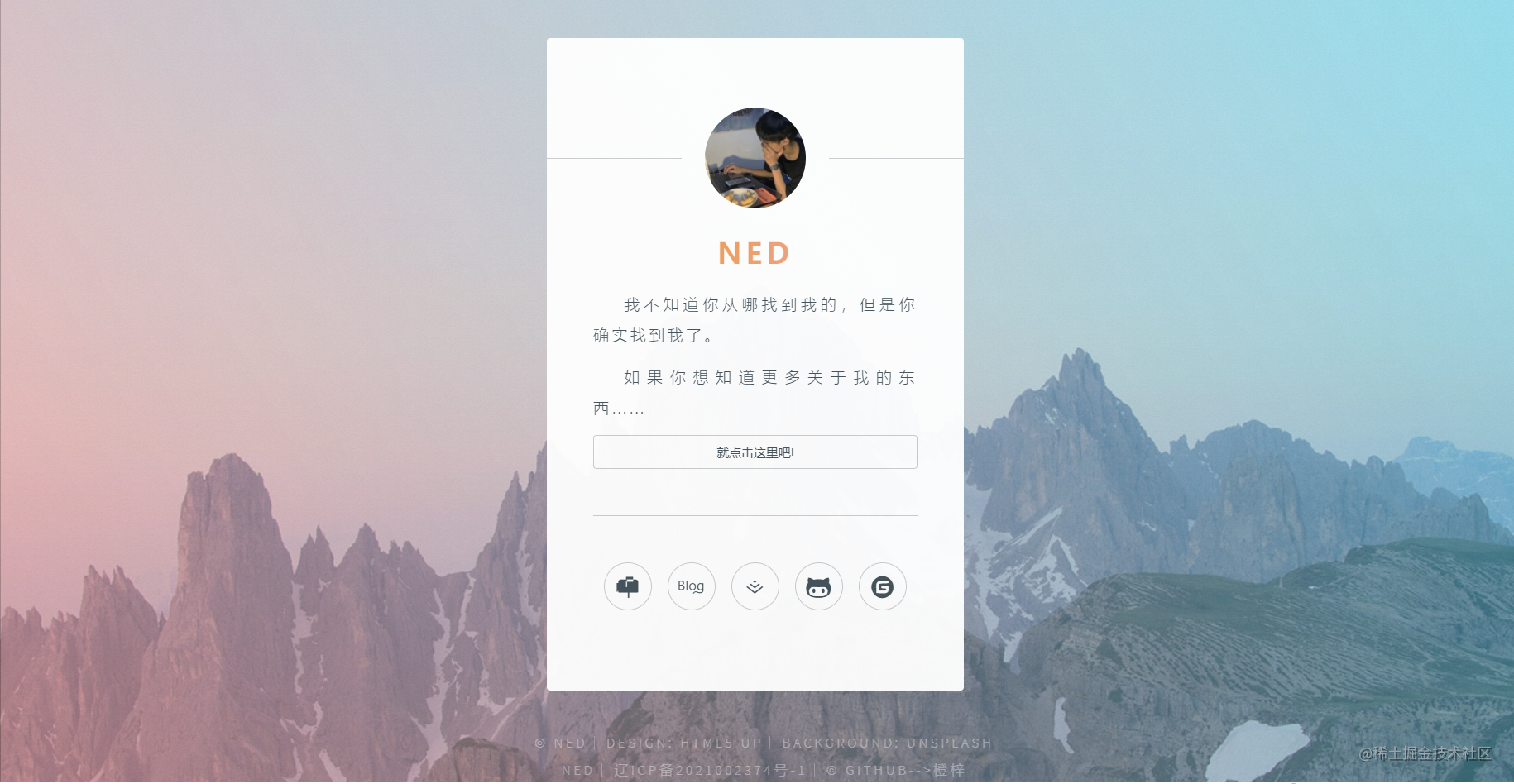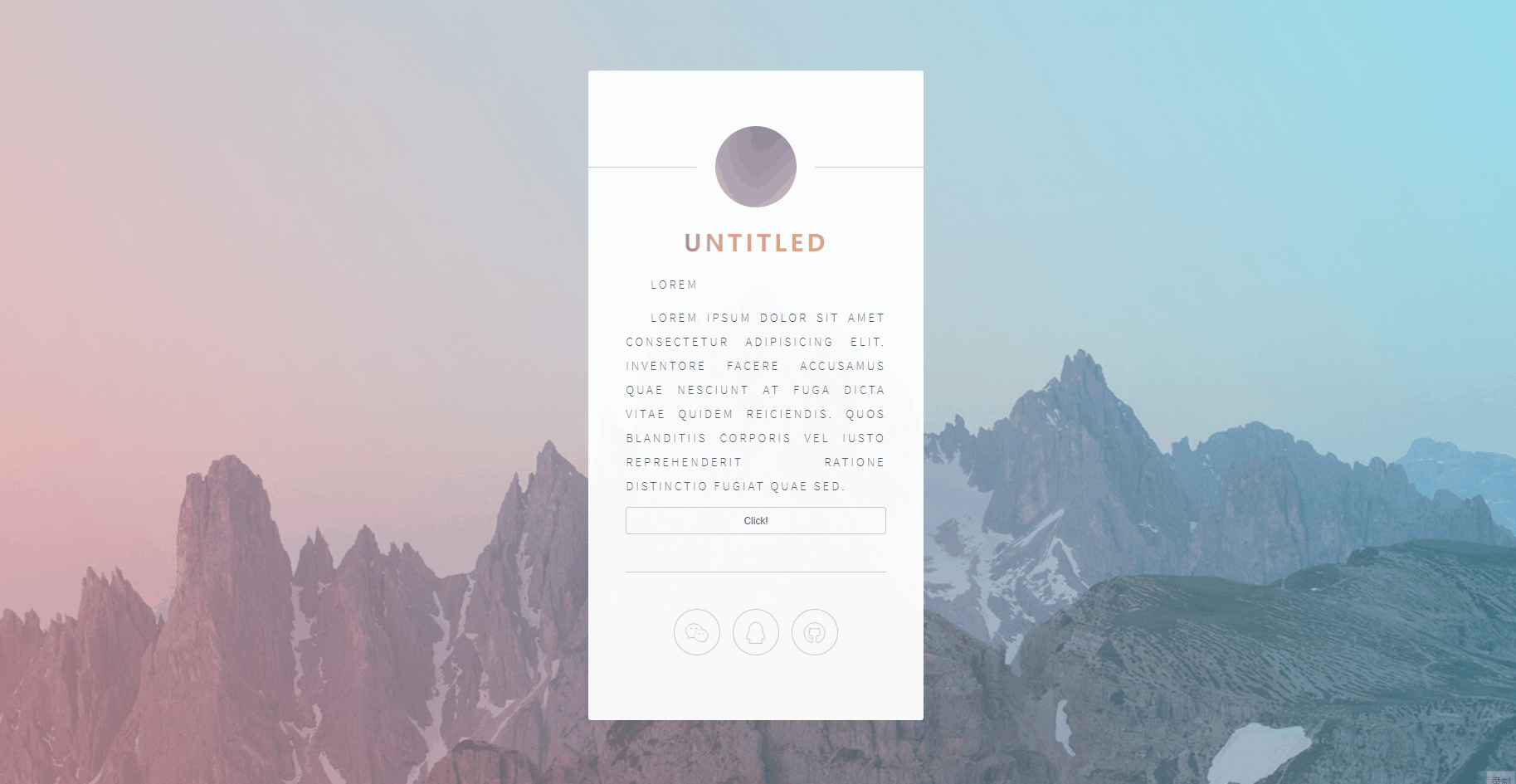我的第一次PR

[
{
name: "Github",
icon: "icon brands fa-github",
desc: "Github",
content: "https://www.wangez.site/",
show: false,
// jump: true,
}
]

showContact: function (name) {
let num = 0;
for (let i = 0; i < this.contacts.length; i++) {
if (this.contacts[i].name == name) {
num = i;
break;
}
}
this.hideContact(false);
this.defaults = 0;
clearTimeout(this.timer);
this.timer = setTimeout(() => {
this.contacts[num].show = true;
this.backButton = true;
}, 500);
}

showContact: function (name) {
let num = 0;
for (let i = 0; i < this.contacts.length; i++) {
if (this.contacts[i].name == name) {
num = i;
break;
}
}
if (this.contacts[num].jump == true) {
window.open(this.contacts[num].content);
return;
}
this.hideContact(false);
this.defaults = 0;
clearTimeout(this.timer);
this.timer = setTimeout(() => {
this.contacts[num].show = true;
this.backButton = true;
}, 500);
}